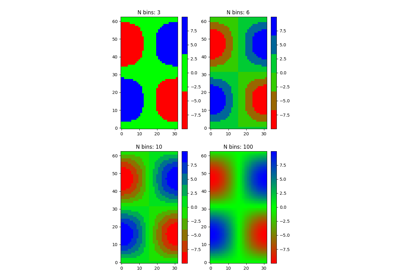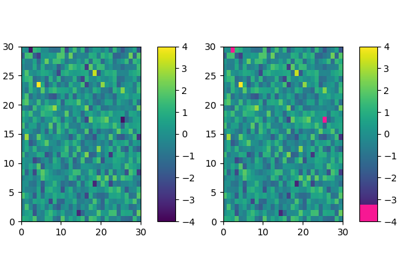# matplotlib.colors.LinearSegmentedColormap¶

class matplotlib.colors.LinearSegmentedColormap(name, segmentdata, N=256, gamma=1.0)[source]

Colormap objects based on lookup tables using linear segments.

The lookup table is generated using linear interpolation for each primary color, with the 0-1 domain divided into any number of segments.

Create color map from linear mapping segments

segmentdata argument is a dictionary with a red, green and blue entries. Each entry should be a list of x, y0, y1 tuples, forming rows in a table. Entries for alpha are optional.

Example: suppose you want red to increase from 0 to 1 over the bottom half, green to do the same over the middle half, and blue over the top half. Then you would use:

cdict = {'red':   [(0.0,  0.0, 0.0),
(0.5,  1.0, 1.0),
(1.0,  1.0, 1.0)],

'green': [(0.0,  0.0, 0.0),
(0.25, 0.0, 0.0),
(0.75, 1.0, 1.0),
(1.0,  1.0, 1.0)],

'blue':  [(0.0,  0.0, 0.0),
(0.5,  0.0, 0.0),
(1.0,  1.0, 1.0)]}


Each row in the table for a given color is a sequence of x, y0, y1 tuples. In each sequence, x must increase monotonically from 0 to 1. For any input value z falling between x[i] and x[i+1], the output value of a given color will be linearly interpolated between y1[i] and y0[i+1]:

row i:   x  y0  y1
/
/
row i+1: x  y0  y1


Hence y0 in the first row and y1 in the last row are never used.

LinearSegmentedColormap.from_list() Static method; factory function for generating a smoothly-varying LinearSegmentedColormap.

makeMappingArray() For information about making a mapping array.

static from_list(name, colors, N=256, gamma=1.0)[source]

Make a linear segmented colormap with name from a sequence of colors which evenly transitions from colors at val=0 to colors[-1] at val=1. N is the number of rgb quantization levels. Alternatively, a list of (value, color) tuples can be given to divide the range unevenly.

reversed(name=None)[source]

Make a reversed instance of the Colormap.

Parameters: name : str, optional The name for the reversed colormap. If it's None the name will be the name of the parent colormap + "_r". LinearSegmentedColormap The reversed colormap.
set_gamma(gamma)[source]

Set a new gamma value and regenerate color map.

## Examples using matplotlib.colors.LinearSegmentedColormap¶Creating a colormap from a list of colorsCreating Colormaps in Matplotlib